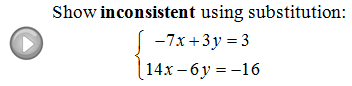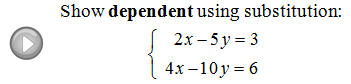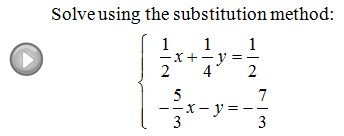## Pages

### Solving Linear Systems by Substitution

The substitution method for solving linear systems is a completely algebraic technique.  There is no need to graph the lines unless you are asked to.  This method is fairly straight forward and always works, the steps are listed below.

Solve the system using the substitution method: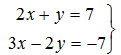Step 1: Use either equation and solve for a variable.In this case, we solved the first equation for y.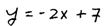Step 2: Substitute the resulting quantity into the other equation.Here we substituted  the quantity found for y into the second equation.Step 3: Solve for the remaining variable.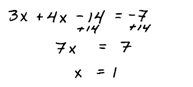Step 4: Back substitute to find the value for the other variable.It does not matter if you choose to solve for x or y first. However, make sure that you do not substitute into the same equation in step 2.

Remember that we are trying to find the simultaneous solutions or the points where the two lines intersect.  Next we will see what happens when the system is dependent, in other words, when the system consists of two lines that are the same.

Solve the system using the substitution method: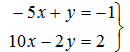Solve for y in the first equation.Any true statement, including 0 = 0, indicates a dependent system.The next system consists of two parallel lines which has no simultaneous solution.

Solve the system using the substitution method: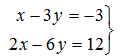Solve for x in the first equation.Any false statement indicates an inconsistent system.Solve the systems using the substitution method.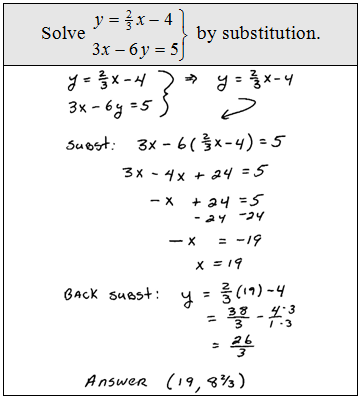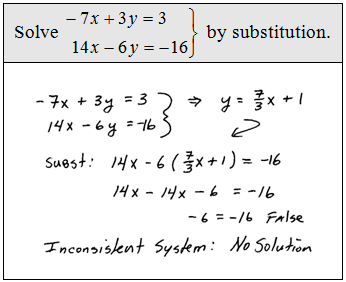Typical word problem: When Joe walked away from the craps table he had 45 chips. He had a combination of \$5 and \$25 chips that added to a total of \$625.  How many of each chip did he have?Set up a system of two linear equations.Solve the system.